# SQRT Excel FunctionArticle byExcelMojo Team## What Is SQRT Function In Excel?

The SQRT function in Excel is an inbuilt Math & Trig function. It calculates the square root of the given number. And the specified number should be positive. Otherwise, the SQRT Excel function will return an error value.

Users can use the SQRT() while performing arithmetic calculations in applications such as hypothesis testing and sample standard deviation evaluation. For example, the table below shows a list of numbers.

And suppose the requirement is to find the square roots of the specified numbers and display the result in column B. Then, we can apply the SQRT() in the target cells and get the required data.

Thus, the SQRT() return value is an integer if the given number is a perfect square. Otherwise, the result is a decimal value.

In the case of fractions, such as cell range A7:A8 data, the values get converted into decimals in the SQRT Excel. And it finds the square root of the decimal numbers, which will be decimal values. But, as the data format of the target cell range B7:B8 is Fraction, we see the output as fractions.

###### Key Takeaways
• The SQRT Excel function computes the square root of the specified positive number. And users can use it while solving complex mathematical equations involving square roots.
• The SQRT function formula is =SQRT(number) and accepts one mandatory argument, number, as input.
• If the given number is negative, use the SQRT function with the ABS function to avoid the SQRT function returning an error value. The formula will, instead, result in the square root of the absolute value of the given negative number.

### SQRT() Excel Formula

The SQRT Excel function syntax is

where,

• number: The number for which we must determine the square root using the SQRT Excel function. And it is a mandatory argument.

Further, below are the critical aspects of the function we must consider to avoid the condition of the SQRT Excel function not working.

• We can directly supply the number as the SQRT function argument. Otherwise, we can provide it as a cell reference in Excel to the number or as a function returning the number.
• If the supplied number argument value is not a number, the SQRT() will throw the #VALUE! error.
• If the supplied number argument value is negative, the SQRT Excel function return value will be the #NUM! error.

### How To Use SQRT Excel Function?

The steps to use the SQRT Excel Function are as follows:

1. First, ensure the given data is a positive number.
2. Then, select the required target cell, and enter the SQRT().
3. Finally, press Enter to view the square root of the specified number.

The example below will explain the above steps in detail to avoid the possibility of the SQRT Excel function not working.

The following table shows a list of numbers.

And suppose the requirement is to determine the square roots of the specified numbers and display them in column B. Then, here is how we can apply the SQRT() in the target cells.

1. Select the target cell B2, enter the SQRT() mentioned in the Formula Bar, and press Enter.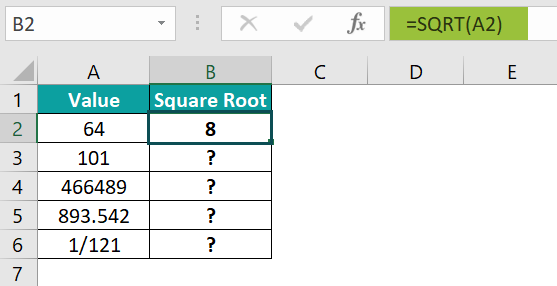On the other hand, the below formula will also result in the same output.
=SQRT(64)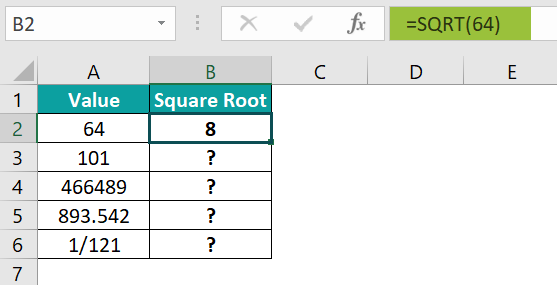Alternatively, we can apply the SQRT() from the Formulas tab.

We can proceed by selecting the target cell B2 and clicking FormulasMath & TrigSQRT to open the Function Arguments window.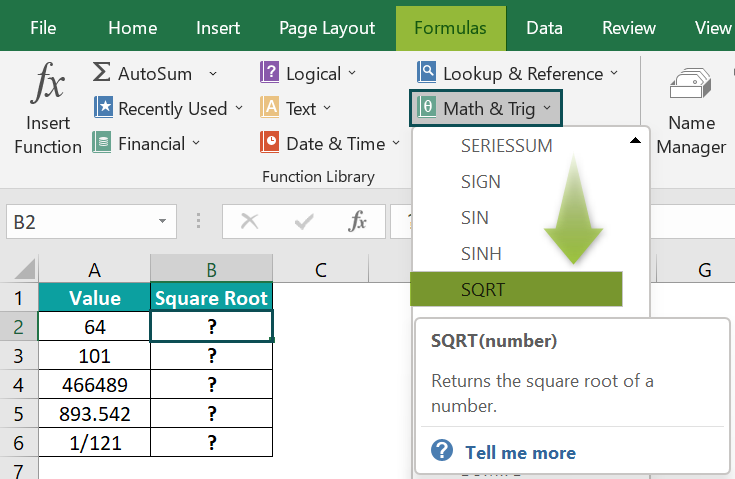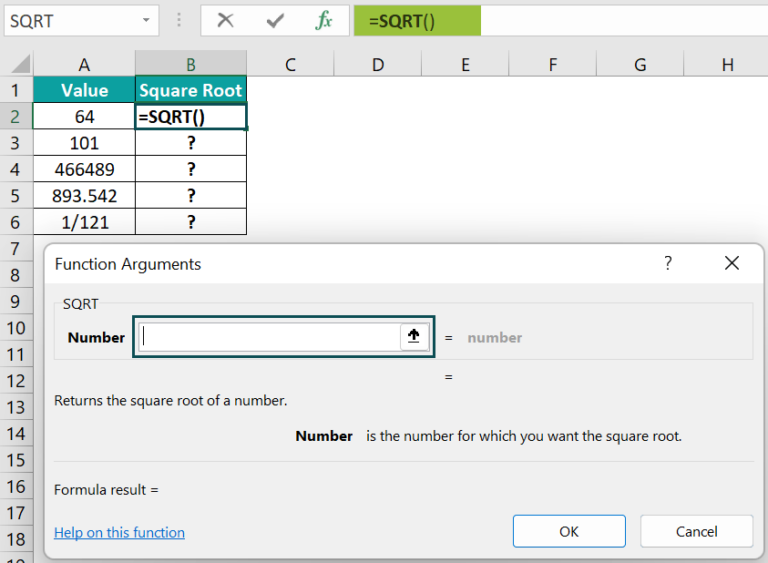Next, enter the specified value in the argument field in the Function Arguments window.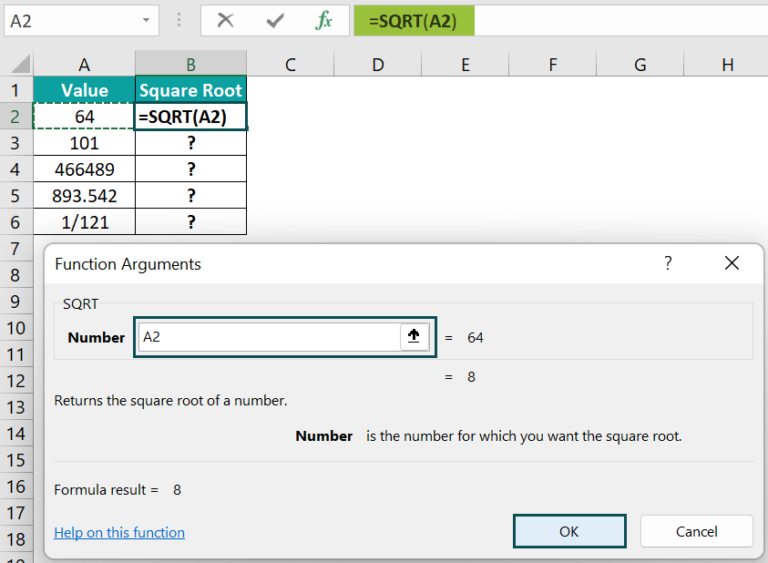And once we click OK in the Function Arguments window, the SQRT() will get executed in the target cell.

2. Copy the formula in cell range B3:B6 using the fill handle.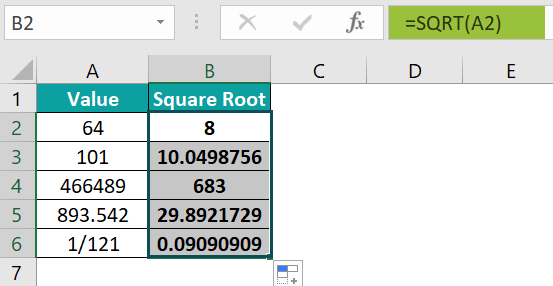3. We need to show the cell B6 output as a fraction. And to do that, select cell B6 and click Home → Number Format → More Number Formats.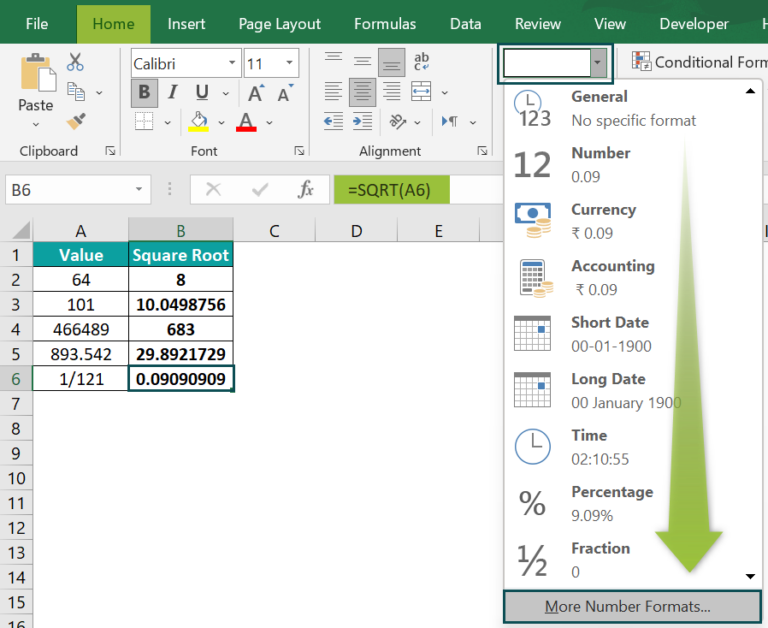The Format Cells window will open.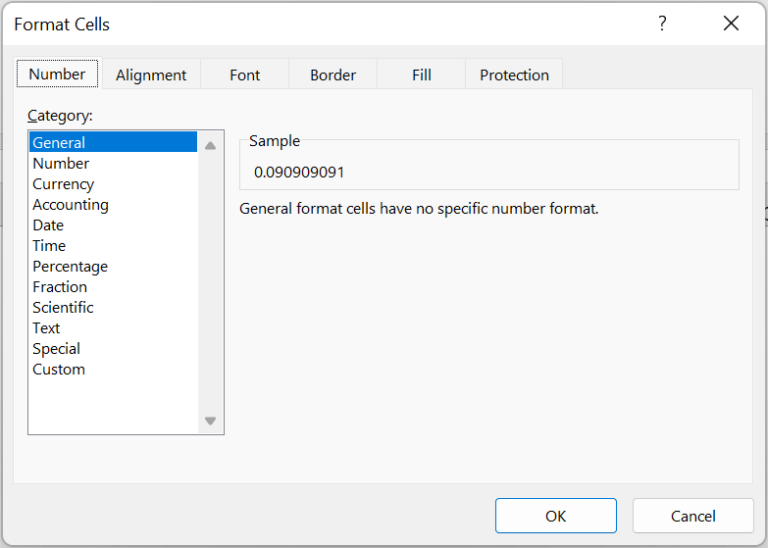In the Number tab, select Fraction and pick the required format.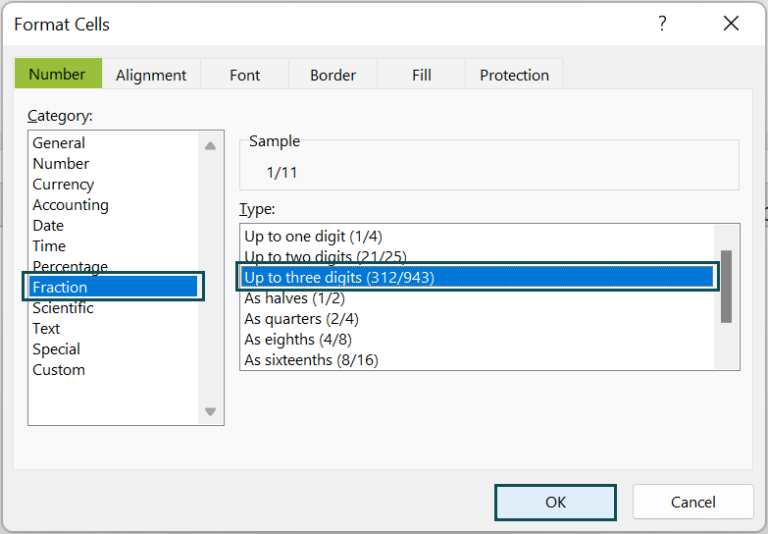And once we click OK in the Format Cells window, the output will be: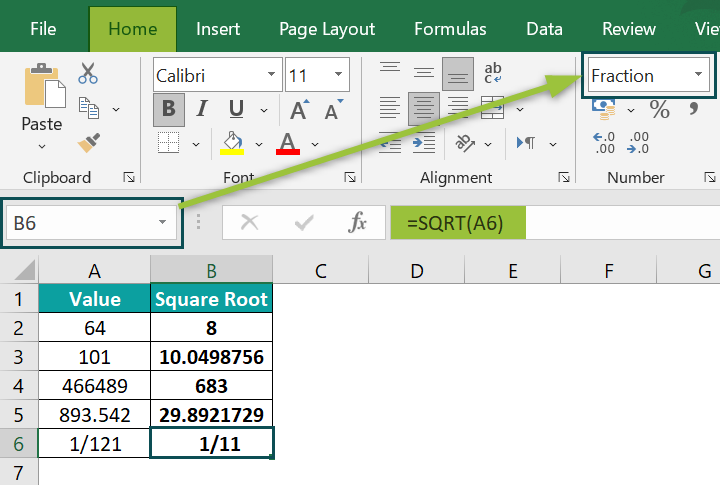### Examples

Check out the following illustrations to understand the SQRT Excel function definition and use the function effectively.

#### Example #1

This example shows SQRT Excel function logic with an equivalent expression.

The table below contains a list of numbers. And we need to calculate their square roots.

We shall now see the working of the SQRT Excel function by applying the required mathematical formula in column B. And then, we shall use the SQRT() in column C to compare the results.

• Step 1: First, select the target cell B2, enter the below formula, and press Enter.

=A2^(1/2)

• Step 2: Next, use the Excel fill handle to copy the expression in cell range B3:B6.

The formula raises the given number to the power of ½ to return the square root of the number.

• Step 3: Select the target cell C2, enter the SQRT Excel function provided in the Formula Bar, and then, press Enter.
• Step 4: Using the fill handle, copy the formula in cell range C3:C6.

Thus, as the mathematical expression returns the same result as the SQRT() in the corresponding target cells, it represents the SQRT Excel function logic.

#### Example #2

Let us see how the SQRT Excel function behaves when the specified number is negative.

Consider the following table containing negative numbers for which we must find the square roots and display the output in the range B2:B4.

• Step 1: To begin with, select the target cell B2, enter the SQRT() provided in the Formula Bar, and press Enter.
• Step 2: Using the fill handle, apply the formula in the range B3:B4.

The SQRT() returns the #NUM! error as a negative number cannot have a real square root since a square is positive or 0.

However, we can apply the SQRT() with the ABS Excel function to find the square root of the negative number’s absolute value.

• Step 3: Select the target cell B2, enter the below formula, and press Enter.

=SQRT(ABS(A2))

• Step 4: Use the fill handle to apply the formula in cell range B3:B4.

The cell B4 formula will return a decimal value. But by setting the cell’s data format as Fraction, as explained in the previous section, we can view the square root as a fraction.

#### Example #3

We can use the SQRT Excel function with other functions, such as IF Excel function.

For example, the table below contains a list of numbers and their assumed square values.

Suppose the requirement is to check if the column B value is the square of the number in the corresponding column A cell. And if the condition holds, display the square root of the column B value in column C.

Then, we can apply the IF() with the SQRT Excel function in the target cells to get the required outcome.

• Step 1: Select the target cell C2, enter the below formula, and then, press Enter.

=IF(A2^2=B2,SQRT(B2),”NA”)

• Step 2: Using the fill handle, copy the formula in the range C3:C6.

Let us consider the cell C6 formula to understand how the formula works. The IF() checks if the square of the cell A6 value equals the cell B6 value. As the condition holds, the SQRT() executes, and the IF() returns the square root of the cell B6 value, 143. Otherwise, the function output would be NA.

### Important Things To Note

• We can provide the SQRT Excel function argument as the specified number, as a cell reference to the number, or as a function that returns the number.
• Remember, the SQRT() argument should always be a number. Otherwise, the function will return the output with the #VALUE! error.
• For a negative number as the argument, the SQRT() will throw the #NUM! error.
• The SQRT function gives fruitful results when used with other inbuilt Excel functions, such as IF, ROUND, and ROUNDDOWN Excel function .

1. How can you apply the SQRT function in Excel VBA?

We can apply the SQRT function in Excel VBA using the below method:

Sqr(number)

The argument number has the same interpretation as explained in the SQRT() Excel Formula section.

The below example shows how we can apply the above method in Excel VBA.
The following table shows a set of numbers.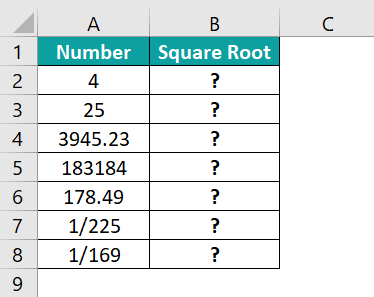And suppose the requirement is to determine the square roots of the given numbers and display the result in column B.

Then applying the SQRT function in Excel VBA can get the required data.

• Step 1: To begin with, open the VBA Editor using the keys Alt + F11.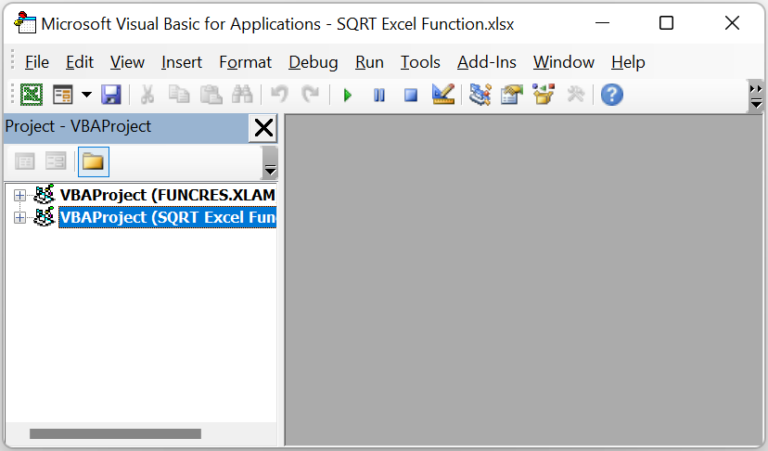• Step 2: Next, choose the required VBAProject and select InsertModule to open a new module, Module1, where we can enter the necessary VBA code.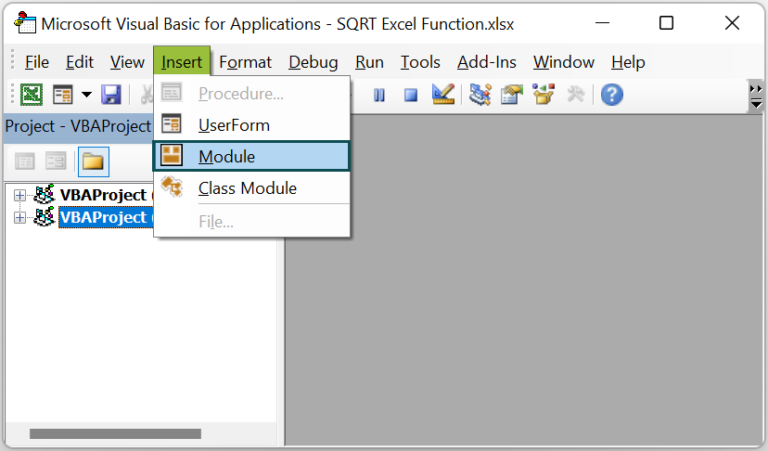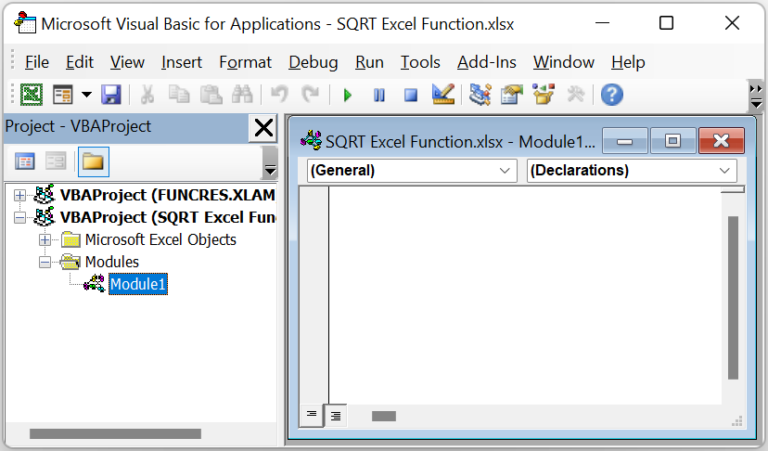• Step 3: Type the VBA code in the Module1 window to apply the SQRT function in the target cells.

Sub SQRT_fn()
Range(“B2”) = Sqr(Range(“A2”))
Range(“B3”) = Sqr(Range(“A3”))
Range(“B4”) = Sqr(Range(“A4”))
Range(“B5”) = Sqr(Range(“A5”))
Range(“B6”) = Sqr(Range(“A6”))
Range(“B7”) = Sqr(Range(“A7”))
Range(“B8”) = Sqr(Range(“A8”))
End Sub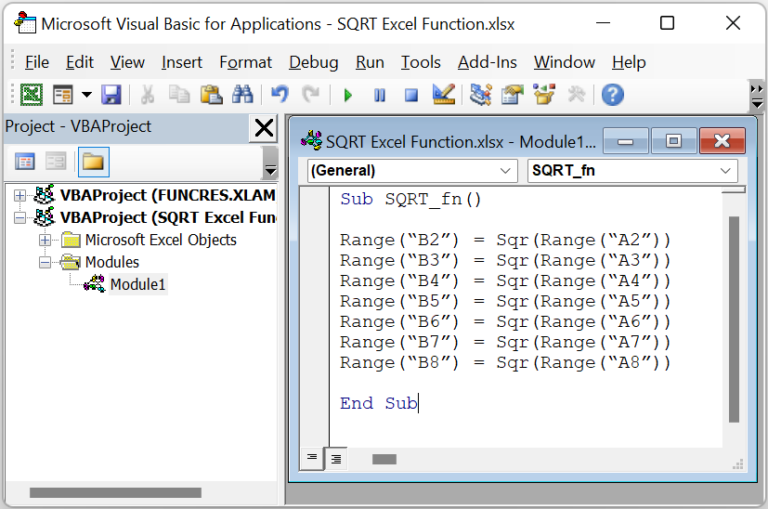• Step 4: Click the Run Sub/UserForm option in the menu to execute the code.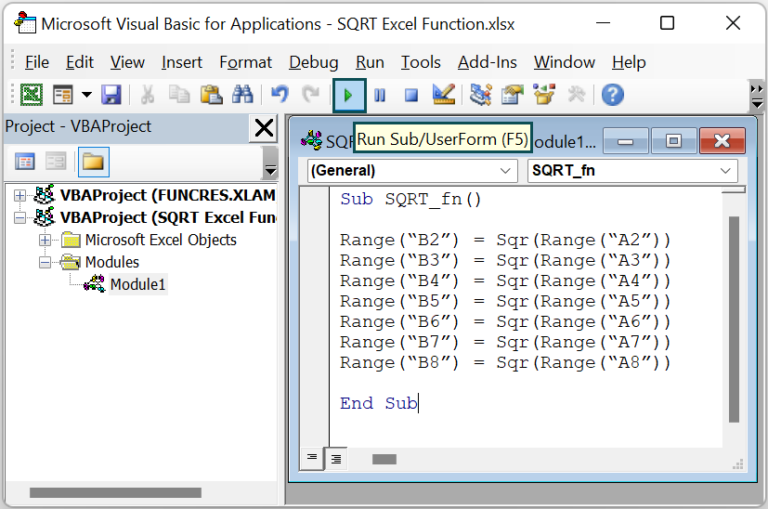We can now open the worksheet containing the source table to see the SQRT function executed in the required target cells.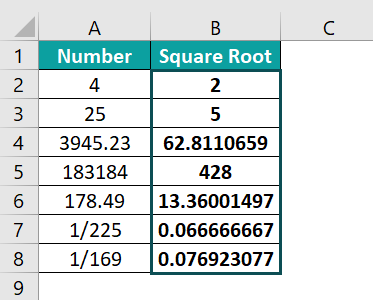Each SQRT method in the VBA code accepts the number specified in the respective column A cell to return the square root of the number in the corresponding target cell in column B.

2. When to use the SQRT Excel function?

We can use the SQRT Excel function to determine the square root of a positive number. And if the specified number is negative, we can apply the SQRT Excel function with the ABS(). The formula will return a positive square root instead of an error value.

3. Why is the SQRT Excel function not working?

The SQRT Excel function may not work, perhaps due to the following reasons:
The supplied SQRT function argument is not a number.
The provided SQRT function argument is a number but a negative value.

4. What is the POWER() formula equivalent to the SQRT Excel function?

The POWER() formula equivalent to the SQRT Excel function is as follows:
=POWER(number,0.5)
Or,
=POWER(number,1/2)
The argument number has the same meaning as explained in the SQRT() Excel Formula section.